# Physics - Classical Mechanics - Simple Harmonic Motion Equations

in STEMGeekslast year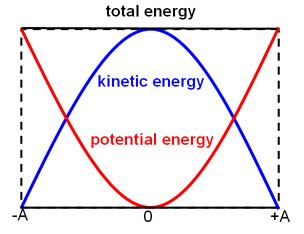[Image1]

## Introduction

Hey it's a me again @drifter1!

Today we continue with Physics, and more specifically the branch of "Classical Mechanics" in order to talk about Simple Harmonic Motion Equations.

So, without further ado, let's get straight into it!

## SHM Equations

In the previous part we approached SHM from the context of energy and showed that the total energy is basically a sum of potential energy and kinetic energy: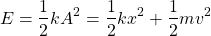Solving this equation for velocity it's easy to end up with: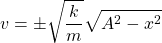For x = 0, where the kinetic energy is maximum, we end up with an equation for the maximum velocity: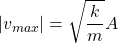### Displacement

That was the easy part. Let's now try to come up with an equation that describes the displacement / position of the oscillator. Of course we have to aim for an equation that gives the displacement in relation to time. That way, we can then easily calculate the velocity and acceleration by simply taking the derivative.

Starting off from the velocity equation, let's replace v with dx/dt, and move everything related to x to one side, and everything related to t to the other side, so that integration is possible. This is known as the variable separation method.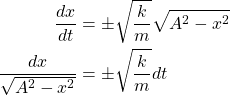Taking the integral now, yields: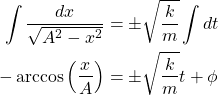where φ is known as the phase angle, and basically shows at which position the oscillation begins (t = 0).

Because cos(-a) = cos(a), we can finally end up with the displacement equation: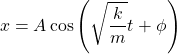Therefore, the position in any SHM is a sinusodial function in relation to time.

The angular frequency, ω, is given by: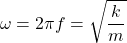and thus we can also write displacement as: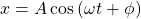### Velocity and Acceleration

Taking the derivative of this last equation, it's easy to come up with equations for velocity and acceleration: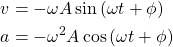### Phase Angle

Let's get more into the various phase angle values now.

When φ = 0, then x0 = A, which is the maximum positive displacement. When φ = π, x0= -A, which is the maximum negative displacement. For φ = π/2 the oscillator starts at x0 = 0.

In general, knowing the initial velocity and position, we can calculate φ as follows: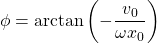This is easy to prove, as it's a simple division of the initial velocity and position equations (set t = 0), which are: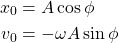followed by an trigonometric equation application.

More on that next time!

### Amplitude

Knowing the initial velocity and position, as well as the angular frequency, it's also possible to calculate the amplitude of the SHM, using: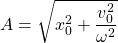Keep that in mind, as it might be useful!

## Previous articles of the series

### Periodic Motion

• Periodic Motion Fundamentals -> Fundamentals (Period, Frequency, Angular Frequency, Return Force, Acceleration, Velocity, Amplitude), Simple Harmonic Motion, Example
• Energy in Simple Harmonic Motion -> Forms of Energy in SHM (Potential, Kinetic, Total and Maximum Energy, Maximum Velocity), Simple Example

## Final words | Next up

And this is actually it for today's post!

Next time we will cover the Reference Circle, which is a nice way of understanding Simple Harmonic Motion...

See ya!Keep on drifting!

Posted with STEMGeeks